Next: Some variants Up: Mathematical characterizations Previous: Relation to principal curves.

#### A decomposition of the cost function.

The cost function of the SOM, Equation 7, can be decomposed into two terms as follows (Lampinen and Oja, 1992; here a discrete version will be presented):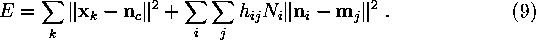Here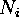denotes the number of the data items which are closest to the reference vector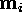, and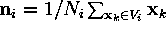, where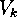is the Voronoi region corresponding to the reference vector. When deriving this approximation is was assumed that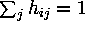for all i, which holds exactly for toroidal maps when the kernel h has the same shape for all i, and also away from the borders on a non-toroidal map if the kernel differs from zero only locally.

The first term in Equation 9 corresponds to the cost function of the K-means clustering algorithm, that is, the average distance from the data points to the nearest cluster centroid. Here the clusters are not defined in terms of their centroids, however, but in terms of the reference vectors. This first term may be interpreted as one way of measuring how accurately the map follows the distribution of the input data.

The second term, on the other hand, may be interpreted as governing the ordering of the reference vectors. In considering the second term it may be of help to note that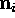andwill in general be close to each other, sinceis the centroid of the cluster defined by. At a fixed point of the SOM algorithm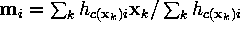, which is closer tothe more the neighborhood kernel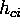is centered around c. To minimize the second term the units that are close to each other on the map should have similar reference vectors, since the value of the neighborhood kernel is large. Units that lie farther away on the map may, on the other hand, have quite dissimilar reference vectors, since the neighborhood kernel is small and the distance thus does not make a large contribution to the error.Next: Some variants Up: Mathematical characterizations Previous: Relation to principal curves.